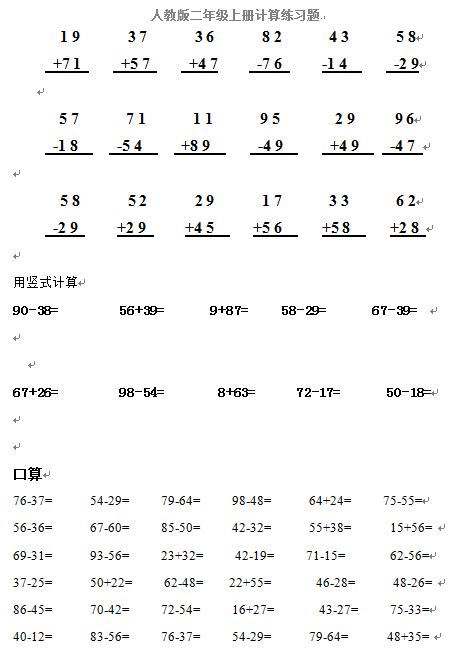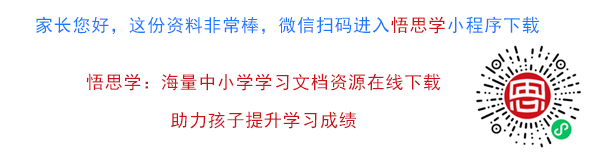90-38=         56+39=       9+87=     58-29=       67-39=

67+26=         98-54=        8+63=      72-17=       50-18=

【DOC文档1页】小学人教版二年级数学上册专项练习：计算题 A4电子版资料_可直接打印_会员免费下载##### 评论信息